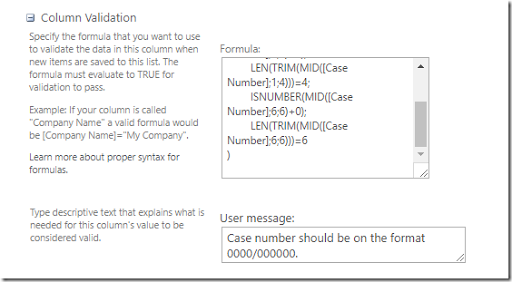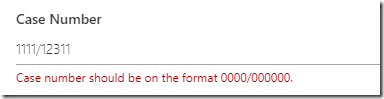## Monday, February 12, 2018

### Validating a number format in SharePoint – divide and conquer formulasPhoto by Oliver Thomas Klein at Unsplash.

Validating input in SharePoint is nothing new, but I haven’t dug much into this over the years. This time around I have a list where one of the fields takes a case number. The case number should be on the format:

0000/000000 <- four digits, a slash, then 6 digits.

Using regular expression this would have been a breeze - \d{4}\/\d{6} , but that’s not an option in SharePoint. Using the official formula documentation as a lookup I ended up with the following formula. I’ve split it over multiple lines and added comments for readability, so remember to remove the comments before using. Depending on your locale you might have to use comma instead of semi-colon.

Make note of the +0 which is workaround to convert text to a number.

```AND(
LEN([Case Number])=11;                # Total length is 11 characters
MID([Case Number];5;1)="/";           # Fifth character is a slash
ISNUMBER(MID([Case Number];1;4)+0);   # First four characters is a number
LEN(TRIM(MID([Case Number];1;4)))=4;  # First four characters has no spaces
ISNUMBER(MID([Case Number];6;6)+0);   # Last six characters is a number
LEN(TRIM(MID([Case Number];6;6)))=6   # Last six characters has no spaces
)
```

Validation is entered at the columns settings.And here I only have 5 digits at the end, which does not validate.